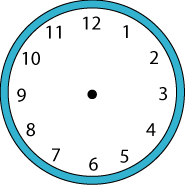#### You may also like### Prompt Cards

These two group activities use mathematical reasoning - one is numerical, one geometric.### Consecutive Numbers

An investigation involving adding and subtracting sets of consecutive numbers. Lots to find out, lots to explore.### Exploring Wild & Wonderful Number Patterns

EWWNP means Exploring Wild and Wonderful Number Patterns Created by Yourself! Investigate what happens if we create number patterns using some simple rules.

# Clock Face

## Clock Face

Can you draw a straight line across the centre of a clock face so that the numbers on both sides of the line have the same total?Can you do this in another way?

Can you draw two lines (like the hands of the clock) to divide the clock face so that the total of the numbers on one side of the lines is twice the total on the other side? Can you do this in another way?

Can you divide the clock face so that that the total on one side of the lines is five times more than the total on the other side? Can you do this in another way?

Can you draw two lines to divide the numbers so that the total of the numbers on each side of the lines are both multiples of six? In how many different ways can you do this?

Can you draw two lines so that the numbers on each side add to a prime number? Can you do this in another way?

Can you find any other interesting ways to group the numbers on a clock face by drawing two lines?

You might find this sheet useful for working on.

### Why do this problem?

This problem requires logical and creative thinking. It is also an intriguing and challenging way to practise addition and subtraction.

### Possible approach

A clock face without hands is a useful introduction for these challenges and it is a good idea to suggest that all the lines are drawn between the numbers rather than from number to number.

Let learners have a go at the first part of the challenge in pairs without saying very much and then after a suitable length of time, bring them together to talk about how they went about finding a solution. Some might have used a trial and improvement approach; others may have found the total of all the numbers on the clock face first and then used some trial and improvement. Discussing strategies at this stage will help children with the other parts of the problem.

You can download this sheet of clock faces to give to the class for rough working, jotting and recording.

### Key questions

What are the totals you are trying to get?
How do you know?
What is the sum of all the numbers on the clock face?

### Possible extension

Pupils could explore other divisions of the clock face and find the time on the clock when the hands do the dividing into different groups of numbers.

### Possible support

You could draw a line across a clock face and ask children to add the numbers on each side to show what is required. Having a calculator available would help if the addition is causing a problem in itself.